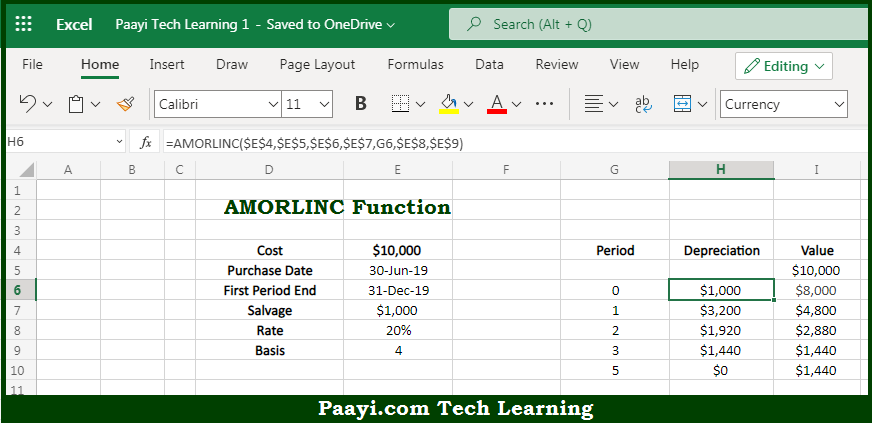# Learn How to Use Microsoft Excel AMORLINC Function

Written by | 0 Comments | 611 Views

In this article, you will learn how to use the Microsoft Excel AMORLINC function and its prime function in Microsoft Excel. You will also get to know the Microsoft Excel AMORLINC function return value and syntax with the help of some examples.

Microsoft Excel AMORLINC Function

The main purpose of the Microsoft Excel AMORLINC function is to get the depreciation for the accounting period. That implies, with the help of the AMORLINC function you can able to return the depreciation for each accounting period. So, with the help of the AMORLINC function, you can able to get the depreciation for the accounting period.

Return Value of AMORLINC Function

The return value will be the depreciation in the given period.

Syntax of AMORLINC Function

=AMORLINC(cost, purchase, first, salvage, period, rate, [basis])

Where the arguments:

• cost: This is the asset cost.
• purchase: This is the asset purchase date.
• first: This is the first-period end date.
• salvage: This is the asset salvage value.
• period: This is the period for which you want to calculate the depreciation.
• rate: This is the rate of depreciation.
• basis: This is the day count basis, default is 0 (optional).

## How to Use Microsoft Excel AMORLINC Function?So we know that Microsoft Excel AMORLINC function you can able to get the depreciation for the accounting period. That implies, with the help of the AMORLINC function you can able to return the depreciation for each accounting period. So, with the help of the AMORLINC function, you can able to get the depreciation for the accounting period.